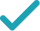Type a math problemSolve for xSteps Using Factoring
Steps Using Factoring By Grouping
Steps for Completing the Square
Steps Using Factoring
Use binomial theorem to expand .
Subtract from to get .
To solve the equation, factor using formula . To find and , set up a system to be solved.
Since is positive, and have the same sign. Since is negative, and are both negative. List all such integer pairs that give product .
Calculate the sum for each pair.
The solution is the pair that gives sum .
Rewrite factored expression using the obtained values.
To find equation solutions, solve and .
GraphGraph Both Sides in 2D
Graph in 2DGiving is as easy as 1, 2, 3
Get 1,000 points to donate to a school of your choice when you join Give With Bing
x^{2}-10x+25-9=0
Use binomial theorem \left(a-b\right)^{2}=a^{2}-2ab+b^{2} to expand \left(x-5\right)^{2}.
x^{2}-10x+16=0
Subtract 9 from 25 to get 16.
a+b=-10 ab=16
To solve the equation, factor x^{2}-10x+16 using formula x^{2}+\left(a+b\right)x+ab=\left(x+a\right)\left(x+b\right). To find a and b, set up a system to be solved.
-1,-16 -2,-8 -4,-4
Since ab is positive, a and b have the same sign. Since a+b is negative, a and b are both negative. List all such integer pairs that give product 16.
-1-16=-17 -2-8=-10 -4-4=-8
Calculate the sum for each pair.
a=-8 b=-2
The solution is the pair that gives sum -10.
\left(x-8\right)\left(x-2\right)
Rewrite factored expression \left(x+a\right)\left(x+b\right) using the obtained values.
x=8 x=2
To find equation solutions, solve x-8=0 and x-2=0.
x^{2}-10x+25-9=0
Use binomial theorem \left(a-b\right)^{2}=a^{2}-2ab+b^{2} to expand \left(x-5\right)^{2}.
x^{2}-10x+16=0
Subtract 9 from 25 to get 16.
a+b=-10 ab=1\times 16=16
To solve the equation, factor the left hand side by grouping. First, left hand side needs to be rewritten as x^{2}+ax+bx+16. To find a and b, set up a system to be solved.
-1,-16 -2,-8 -4,-4
Since ab is positive, a and b have the same sign. Since a+b is negative, a and b are both negative. List all such integer pairs that give product 16.
-1-16=-17 -2-8=-10 -4-4=-8
Calculate the sum for each pair.
a=-8 b=-2
The solution is the pair that gives sum -10.
\left(x^{2}-8x\right)+\left(-2x+16\right)
Rewrite x^{2}-10x+16 as \left(x^{2}-8x\right)+\left(-2x+16\right).
x\left(x-8\right)-2\left(x-8\right)
Factor out x in the first and -2 in the second group.
\left(x-8\right)\left(x-2\right)
Factor out common term x-8 by using distributive property.
x=8 x=2
To find equation solutions, solve x-8=0 and x-2=0.
x^{2}-10x+25-9=0
Use binomial theorem \left(a-b\right)^{2}=a^{2}-2ab+b^{2} to expand \left(x-5\right)^{2}.
x^{2}-10x+16=0
Subtract 9 from 25 to get 16.
x=\frac{-\left(-10\right)±\sqrt{\left(-10\right)^{2}-4\times 16}}{2}
This equation is in standard form: ax^{2}+bx+c=0. Substitute 1 for a, -10 for b, and 16 for c in the quadratic formula, \frac{-b±\sqrt{b^{2}-4ac}}{2a}.
x=\frac{-\left(-10\right)±\sqrt{100-4\times 16}}{2}
Square -10.
x=\frac{-\left(-10\right)±\sqrt{100-64}}{2}
Multiply -4 times 16.
x=\frac{-\left(-10\right)±\sqrt{36}}{2}
x=\frac{-\left(-10\right)±6}{2}
Take the square root of 36.
x=\frac{10±6}{2}
The opposite of -10 is 10.
x=\frac{16}{2}
Now solve the equation x=\frac{10±6}{2} when ± is plus. Add 10 to 6.
x=8
Divide 16 by 2.
x=\frac{4}{2}
Now solve the equation x=\frac{10±6}{2} when ± is minus. Subtract 6 from 10.
x=2
Divide 4 by 2.
x=8 x=2
The equation is now solved.
x^{2}-10x+25-9=0
Use binomial theorem \left(a-b\right)^{2}=a^{2}-2ab+b^{2} to expand \left(x-5\right)^{2}.
x^{2}-10x+16=0
Subtract 9 from 25 to get 16.
x^{2}-10x=-16
Subtract 16 from both sides. Anything subtracted from zero gives its negation.
x^{2}-10x+\left(-5\right)^{2}=-16+\left(-5\right)^{2}
Divide -10, the coefficient of the x term, by 2 to get -5. Then add the square of -5 to both sides of the equation. This step makes the left hand side of the equation a perfect square.
x^{2}-10x+25=-16+25
Square -5.
x^{2}-10x+25=9